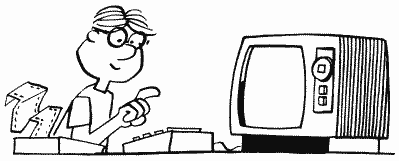DECIMAL TO HEXLet's take the number 9304. Look in the chart to find the largest number that is SMALLER than 9304. That would be 8192, which is the second number in the fourth column. This means the hex humber will have four digits because you found the decimal value 8192 in the fourth column. Write down the "hex #" from the chart in the fourth place:

2---

Now subtract 8192 from the original number of 9304 to leave a remainder of 1112. Looking at the chart again we find the nearest SMALLER number of 1024 in the third column. Put down the corresponding hex # and subtract 1024 from 1112:

24--

Do the same for the new remainder of 88 to find the hex # digit for the second and first place of the hex number. Don't forget to mark hex numbers with a "\$" as is standard with computer types like us. Here is the complete example (Example 1):

Decimal-to-Hex

9304
-8192 (largest # less than 9304 on chart, 4th column) ----→ 2
1112
-1024 3rd column -----------------------------------------------→ 4
88
80 2nd column ----------------------------------------------→ 5
8 1st column -----------------------------------------------→ 8 = \$2458

Example 1. Decimal-to-hex example

If you find that you have numbers for only the first and third places, this means to add a 0 as a placeholder. \$040F would be an example.